# Python: A Whirlwind Tour¶

## A small Introduction¶

The purpose of this document is to teach/remind you how Python works. Throughout this tutorial there are links for further resources and websites, just in case you are not satisfied with how a particular concept is explained.

Below is a list of websites that have some Python tutorials:

Remember, if you are stuck, then you can usually Google the issue and find other people who have had a similar problem. StackOverflow is a great question and answer site for programming, so if you see it on Google, it's usually a good result to look at.

## A short overview on how programs work¶

A program is a list of instructions that are executed, line-by-line by a computer. The instructions are written in a programming language and are converted into binary for the computer to understand.

We are going to be using Python as it is easy to learn and there is an abundance of useful libraries (pre-written code).

To write your own program, you will be using a code editor. Python comes with its own editor called IDLE, but we will use Visual Studio Code (VSCode) instead, as it has some built-in features that should make programming easier.

Open 'Visual Studio Code' (Not 'Visual Studio') on your machine and create a new file. When prompted which language to use, choose 'Python'. If you're prompted to install the recommended python extension, go ahead and click install.

## Variables¶

In a program you often need to store information to use later; to do this you would use a variable.

Variables are called that because the value stored in one can change, or vary. Some languages have constants whose values can’t change but these don’t exist in Python.

To assign a value to a variable in Python we specify a name for the variable, followed by the `=`sign, followed by the value e.g:

``````name = "Mary"
age = 17
``````

A variable could store many types of data including numbers, text, collections of numbers or text, entire robots and much more.

Here are some of the built-in types:

Name of type Example
String `"Hello World"`
Integer `6`
Float `4.2`
Boolean `True`
List `[1, 2, 3, 4, 5, 6]`
Dictionary `{"a": 1, "b": 2}`

In Python you don’t need to tell the computer what type your variable is, the computer will just work it out based on the value you assign to it. It’s generally frowned upon to change a variable’s value to a different type, although Python does let you do it.

The print statement displays what it’s given in the output window. This is useful for debugging as you can use it to see what value a variable is storing. For example:

``````print("Hello world")
``````

Would output the following in the console:

``````Hello world
``````

We can also print variables. Here, variable `a` stores the number `4.2`:

``````>>> a = 4.2
>>> print(a)
4.2
``````

## Input Statement¶

`input()` is the opposite of `print()`. It lets you type something in and get it as a variable. Here are a few examples:

``````>>> a = input()
Hello
>>> print(a)
Hello
``````

As you can see, the program waits for you to give an input which is Hello in this example. When you print `a` it outputs the string Hello - which is what was inputted.

`input()` always returns a string, so If you want to input numbers (integers or floats) then you will need to convert the input to the appropriate variable type using the `int()` or `float()` functions.

``````a = int(input())
``````

While you won’t be able to use `input()` in the competition (the robots don’t have keyboards), it can be quite useful for practising or for testing ideas.

## Commenting¶

Making the intent of your code clear is very important, even more so when working in a team. Use comments to explain why the code is behaving a certain way without affecting the program flow. Any text after a ‘#’ will be ignored e.g:

``````# A comment on its own line
favourite_insect = "Bee"  # Bees are cool. (Comment on same line as code)
``````

## Data Types¶

### Storing text - Strings¶

Strings are used to store a bunch of characters. To create a string, put speech marks ("") around the text that you want to be in the string. For example:

``````>>> a = "Foo"
>>> a
'Foo'
>>> "abc" + "def"  # Concatenate 2 strings together
'abcdef'
>>> str(42)  # Convert something else to a string
'42'
``````

### Storing numbers - Integers and decimals¶

As the name implies, integer variables store integers (whole numbers). In Python decimals (known as floats) and integers can be used interchangeably and will work with each other without you having to think about it.

``````bees = 100
honey = 0.3
total_honey = honey * bees  # evaluates to 30
``````

Operations involving numbers are covered in the Doing Maths in Python section.

### Storing Collections - Lists¶

Lists are a bunch of values under one variable. They are defined by putting the values within square brackets and separating the variables with commas, like this:

``````a  =  [1, 2, 3, 4, 5, 6, 7]
``````

Get certain items from a list using the list index. The index starts at 0:

``````a  # Evaluates to 1
a[-1]  # Evaluates to 7 - negative numbers count backwards from the end
``````

As it so happens, strings are lists of characters! You can use list operations on them too:

``````alpha = "abcdefg"
letter = alpha  # letter is "e"
cabbage = alpha + alpha + alpha*2 + alpha + alpha + alpha
``````

### True / False logic - Booleans¶

Boolean variables can only be `True` or `False`. Booleans are used for conditions in `if` statements, `while` loops and `for` loops. These will come up later.

You can use boolean operators to make complex decisions based on lots of variables.

Symbol/Operator Description Example that would be `True` Example that would be `False`
`==` Equals `1 == 1` `1 == 2`
`!=` Does not equal `1 != 2` `1 != 1`
`>` Greater Than `4 > 2` `3 > 4`
`<` Less Than `2 < 4` `4 > 1`
>= Greater Than or Equal To `4 >= 4` `4 >= 6`
<= Less Than or Equal To `2 <= 2` `7 >= 1`
`and` Logical 'and' operator `True and True` `False and False`
`or` Logical 'or' operator `True or False` `False or False`
`not` Logical 'not' operator `not False` `not True`

## Using Logic¶

### If Statements¶

If statements let you execute code depending on whether the condition evaluates to true, e.g:

``````name = "mary"
if len(name) > 3:
print("Your name isn't all that short")
``````

You can add an `else` statement following your `if` statement to execute code if the condition is false, e.g:

``````name = "mary"
if len(name) > 3:
print("Your name isn't all that short")
else:
print("Wow that's a short name")
``````

If you want to chain if statements together you can use the `elif` statement like so:

``````name = "mary"
if len(name) > 3:
print("Your name isn't all that short")
elif len(name) > 10:
print("Wow that's a long name")
else:
print("Wow that's a short name")
``````

### While Loops¶

A `while` loop will continue executing the code within it while the condition is true, e.g:

``````while True:
print("I know a song that will get on your nerves")
print("I know a song that will get on your nerves")
print("And this is how it goes")
``````

In order to not get ‘stuck’ in a while loop you should make sure that you change the condition within the loop:

``````number_of_sheep = 0
while number_of_sheep < 10:
print("Hope I sleep soon")
number_of_sheep += 1
print("zzzzzzzzz")
``````

### For Loops¶

An `for` loop and an `in` statement lets you loop through each item in a list, e.g:

``````tasty_types_of_cheese = ["Cheddar", "Camembert", "Gouda", "Brie"]
for cheese in tasty_types_of_cheese:
print(cheese + " is tasty")
``````

### Indentation¶

Indentation is important in Python. For code to be counted as part of a loop, `if` statement or function it has to be indented properly. The standard indent size is 4 spaces. Pressing ‘tab’ in VSCode will automatically indent the configured amount.

``````tasty_types_of_cheese = ["Cheddar", "Camembert", "Gouda", "Brie"]
for cheese in tasty_types_of_cheese:
print(cheese + " is tasty")  # Part of the loop
print("mmm yum")  # Not indented so not part of the loop
``````

## Doing Maths in Python¶

### Basic Operators¶

Maths in Python is very similar to the way we do maths in a calculator. The order of operations follows BIDMAS (Brackets, Indices, Division, Multiplication, Addition and Subtraction). We would recommend using a liberal amount of brackets to ensure that the correct order of operations is carried out.

You can also use variables when doing mathematical operations, however they need to be floats or integers. Mathematical operations won’t always work on strings or booleans.

Below is a list of mathematical operators that you can use:

Operator Name in Python Operator in mathematical form
Add `a + b`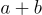Subtract `a - b`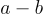Divide `a / b`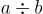Multiply `a * b`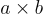Powers `a ** b`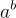### Using the `math` module¶

The `math` module has several mathematical functions that could be useful for robot development (e.g trigonometry functions). To access this module, it must be imported.

``````>>> import math
>>> math.factorial(5)
120
>>> math.cos(0)
1.0
``````

## Debugging (and better print statements)¶

Sometimes you might come across issues with your code that you may need to debug. The `print()` statement comes in handy in these situations. You might find it useful to insert `print()` statements at various points in your code to track the values of variables:

``````x = 40
print("initialised x as 40")
x = x + 2
print("x is now:", x)
``````

### f-strings¶

For inserting values into strings we recommend f-strings. f-strings look like normal strings, but with an f in front like this: `f"This is an f-string"`. Unlike normal strings, f-strings let you insert values into them inside curly brackets {} like this:

``````var_age = 20
print(f"my age is {var_age}"))  # Prints "my age is 20"
``````

You can put any valid value in the curly brackets:

``````f"{["Mammal", "Reptile", "Fish", "Amphibian", "Bird"]} is the word"
``````

## Functions¶

Functions are named blocks of reusable code. They’re useful for making your code neater and easier to read, which is important as you don’t have that much time to debug errors. Using functions reduces the amount of repeated code in your program. Make them like this:

``````def function_name():
print("This function will print this string")
``````

This function prints `"This function will print this string"` every time it’s called.

``````>>> function_name()
This function will print this string
``````

Functions can take in extra data called parameters. These work like variables that you can only access from within the function, and their values get set when the function gets called.

``````def turn_right(angle, speed):
print(f"set the left motor to {speed}")
print(f"set the right motor to {-speed}")
print(f"wait {angle / 100} seconds")
print("set the left motor to 0")
print("set the right motor to 0")
``````

Here’s what happens when you call `turn_right`:

``````>>> turn_right(45, 0.7)
set the left motor to 0.7
set the right motor to -0.7
wait 0.45 seconds
set the left motor to 0
set the right motor to 0
``````

Functions can also return values, which can then be stored in variables, passed to other functions or even just ignored. Here’s a function which returns a value:

``````import math
return degrees * math.pi / 180
``````

And here’s that function in action:

``````>>> radians = degrees_to_radians(360)
6.283185307179586
``````

## Scope of a variable¶

Not all variables are accessible in every part of the code. Where a variable can be accessed from is called its scope. If the variable is defined in a function, then you can only use that variable inside the same function. Variables defined outside functions can be used anywhere.

If you want a variable in a function to be accessible everywhere, write `global` in front of its name when you define it (e.g `global myVar = 2`). You shouldn’t usually need to do this, though.

Start by importing the module you want with the statement `import module_name`. You can then use its functions by writing `module_name.function()`. For example, if you wanted to use the `sleep` function in the `time` module then you would write `time.sleep()`.
Some module names are quite long, so you can shorten the code by assigning it a different name when you import it by using the `as` keyword. For example, `matplotlib.pyplot` allows you to plot graphs, but you can shorten it to “plt” in your code by writing this when you import it: `import matplotlib.pyplot as plt`.
Any other Python files in the same folder can be imported like modules. If you have a file called `motor_control.py`, for example, you could import it by writing `import motor_control`.